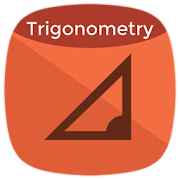# Trigonometry - Lumos Educational App Store4.31
Price -fREE
\$0

#### DESCRIPTION:

This app has two section, first one is a complete trigonometric calculator and another is a complete list of trigonometric identities and formulas. â¢ Trigonometric Calculator: Calculate Sine Function (sin), Cosine Function (cos), Tangent Function (tan), Cotangent Function (cot), Cosecant Function (csc) and Secant Function (sec) of angle â¢ Inverse Trigonometric Calculator: Calculate Inverse Sine Function (arcsin), Inverse Cosine Function (arccos), Inverse Tangent Function (arctan), Inverse Cotangent Function (arccot), Inverse Cosecant Function (arccsc) and Inverse Secant Function

#### OVERVIEW:

Trigonometry is a free educational mobile app By .It helps students in grades HS practice the following standards HSF.TF.C.8.

This page not only allows students and teachers download Trigonometry but also find engaging Sample Questions, Videos, Pins, Worksheets, Books related to the following topics.

1. HSF.TF.C.8 : Prove the Pythagorean identity sin2(θ) + cos2(θ) = 1 and use it to find sin(θ), cos(θ), or tan(θ) given sin(θ), cos(θ), or tan(θ) and the quadrant of the angle..

HS

#### STANDARDS:

HSF.TF.C.8

Developer:

Software Version: 3.0.1

Category:

### RELATED APPSEdSearch WebSearch